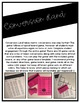# Metric Measurement Conversion: Conversion Chart and GamesSubject
Resource Type
File Type

PDF

(18 MB|32 pages)
Standards
• Product Description
• StandardsNEW
This Metric Measurement Product is all inclusive! Included is a Metric Conversion Chart display that uses the pneumonic "King Henry Doesn't Usually Drink Chocolate Milk" to help students remember the order of the metric prefixes. Add in the arrows and the multiple or divide by 10 signs and students will have this whole metric conversions thing down in no time!

In addition to the classroom display of the conversion chart is a student desktop version of the metric conversion chart that uses the familiar language to aide in students understanding.

Following the conversion charts are TWO metric conversion games. The first game is a "Find Someone Who..." game. In this game, students get up and moving while solving metric conversions. Make it a race and you have instant engagement. To play this game, students will find someone in the classroom that can solve one of the metric conversions on their sheet. Students will trade papers and solve one conversion on each others paper. The student with their entire sheet filled correctly first-takes the cake!

The second game included follows the format of a traditional board game. However, in this game- all students must solve ALL equations. No turns, no outs! All students are engaged throughout the entirety of the game. In order for a student to move their pawn, all players must agree that the players answer is correct. There are 40 playing cards included- 22 of which are word problems for metric conversion.

I hope that your students enjoy this product as much as my students do!

Common Core Standards: 4.MD.1 and 4.MD.2
Convert among different-sized standard measurement units within a given measurement system (e.g., convert 5 cm to 0.05 m), and use these conversions in solving multi-step, real world problems.
Use the four operations to solve word problems involving distances, intervals of time, liquid volumes, masses of objects, and money, including problems involving simple fractions or decimals, and problems that require expressing measurements given in a larger unit in terms of a smaller unit. Represent measurement quantities using diagrams such as number line diagrams that feature a measurement scale.
Know relative sizes of measurement units within one system of units including km, m, cm; kg, g; lb, oz.; l, ml; hr, min, sec. Within a single system of measurement, express measurements in a larger unit in terms of a smaller unit. Record measurement equivalents in a two-column table. For example, know that 1 ft is 12 times as long as 1 in. Express the length of a 4 ft snake as 48 in. Generate a conversion table for feet and inches listing the number pairs (1, 12), (2, 24), (3, 36),...
Measure and estimate liquid volumes and masses of objects using standard units of grams (g), kilograms (kg), and liters (l). Add, subtract, multiply, or divide to solve one-step word problems involving masses or volumes that are given in the same units, e.g., by using drawings (such as a beaker with a measurement scale) to represent the problem.
Total Pages
32 pages
Included
Teaching Duration
N/A
Report this Resource to TpT
Reported resources will be reviewed by our team. Report this resource to let us know if this resource violates TpT’s content guidelines.